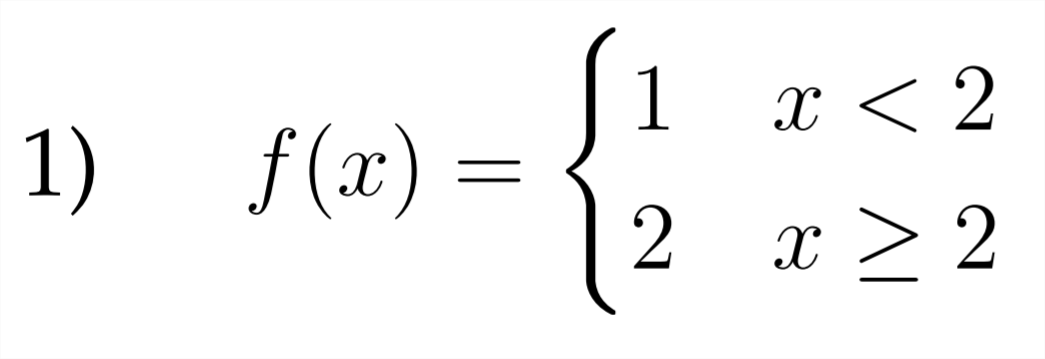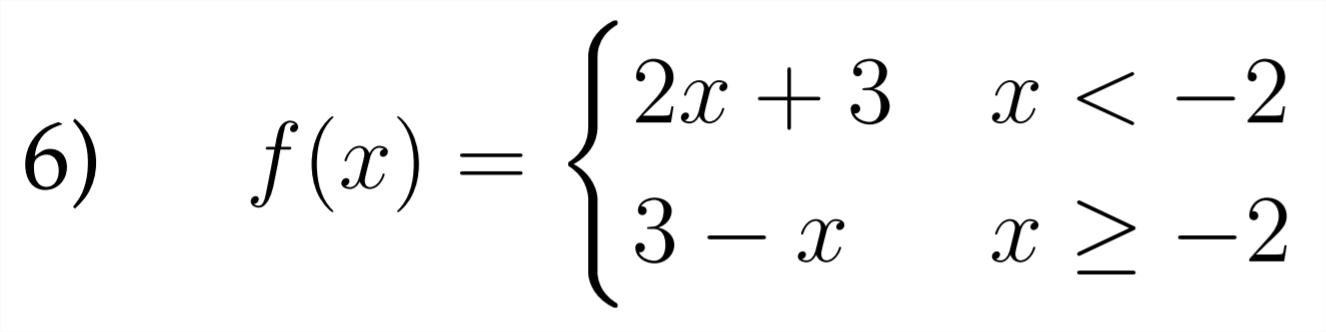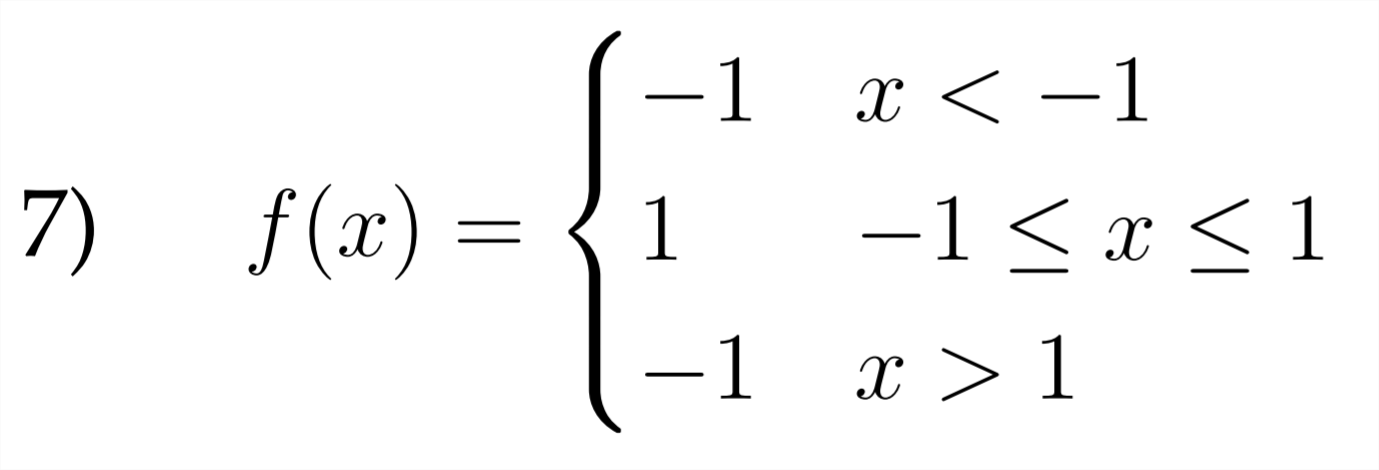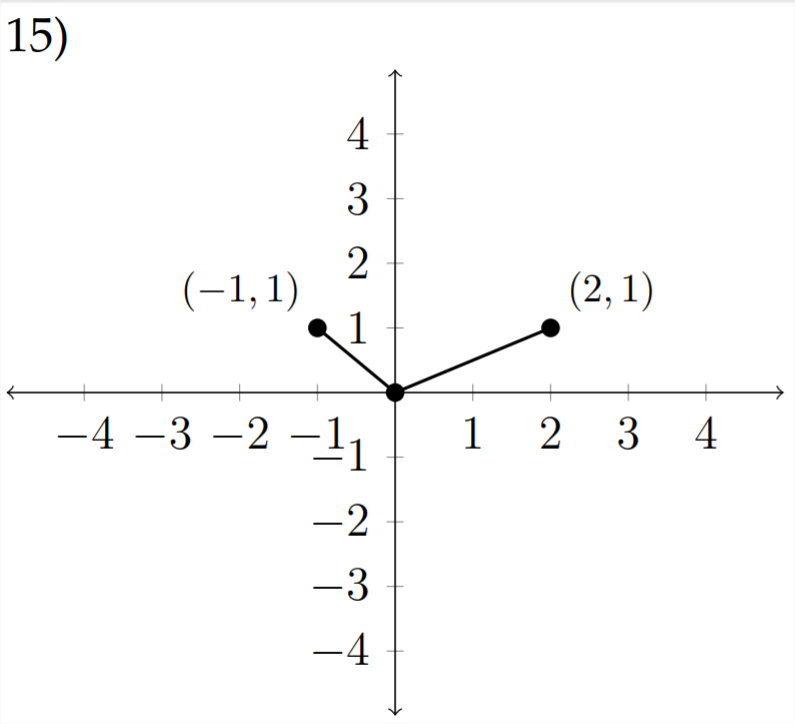# 4.6: Piecewise-defined Functions

$$\newcommand{\vecs}{\overset { \rightharpoonup} {\mathbf{#1}} }$$ $$\newcommand{\vecd}{\overset{-\!-\!\rightharpoonup}{\vphantom{a}\smash {#1}}}$$$$\newcommand{\id}{\mathrm{id}}$$ $$\newcommand{\Span}{\mathrm{span}}$$ $$\newcommand{\kernel}{\mathrm{null}\,}$$ $$\newcommand{\range}{\mathrm{range}\,}$$ $$\newcommand{\RealPart}{\mathrm{Re}}$$ $$\newcommand{\ImaginaryPart}{\mathrm{Im}}$$ $$\newcommand{\Argument}{\mathrm{Arg}}$$ $$\newcommand{\norm}{\| #1 \|}$$ $$\newcommand{\inner}{\langle #1, #2 \rangle}$$ $$\newcommand{\Span}{\mathrm{span}}$$ $$\newcommand{\id}{\mathrm{id}}$$ $$\newcommand{\Span}{\mathrm{span}}$$ $$\newcommand{\kernel}{\mathrm{null}\,}$$ $$\newcommand{\range}{\mathrm{range}\,}$$ $$\newcommand{\RealPart}{\mathrm{Re}}$$ $$\newcommand{\ImaginaryPart}{\mathrm{Im}}$$ $$\newcommand{\Argument}{\mathrm{Arg}}$$ $$\newcommand{\norm}{\| #1 \|}$$ $$\newcommand{\inner}{\langle #1, #2 \rangle}$$ $$\newcommand{\Span}{\mathrm{span}}$$$$\newcommand{\AA}{\unicode[.8,0]{x212B}}$$

In certain situations a numerical relationship may follow one pattern of behavior for a while and then exhibit a different kind of behavior. In a situation such as this, it is helpful to use what is known as a piecewise defined function - a function that is defined in pieces.In the above example of a piecewise defined function, we see that the $$y$$ values for the negative values of $$x$$ are defined differently than the $$y$$ values for the positive values of $$x$$Sometimes we are given a graph and need to write a piecewise description of the function it describes.The piecewise function pictured above could be described as follows:Exercises 4.6
Sketch a graph for each of the piecewise functions described below.This page titled 4.6: Piecewise-defined Functions is shared under a CC BY-NC-SA 4.0 license and was authored, remixed, and/or curated by Richard W. Beveridge.